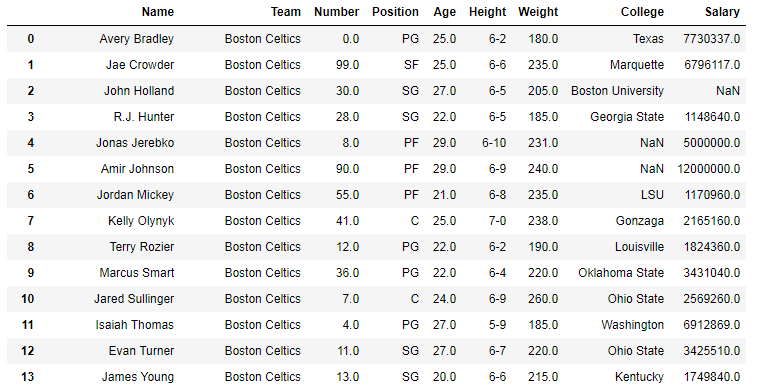# Python | Pandas df.size, df.shape and df.ndim

• Last Updated : 03 Oct, 2018

Python is a great language for doing data analysis, primarily because of the fantastic ecosystem of data-centric Python packages. Pandas is one of those packages and makes importing and analyzing data much easier.

Pandas `.size`, `.shape` and `.ndim` are used to return size, shape and dimensions of data frames and series.

Syntax: dataframe.size
Return : Returns size of dataframe/series which is equivalent to total number of elements. That is rows x columns.

Syntax: dataframe.shape
Return : Returns tuple of shape (Rows, columns) of dataframe/series

Syntax: dataframe.ndim
Return : Returns dimension of dataframe/series. 1 for one dimension (series), 2 for two dimension (dataframe)

In the following examples, the data frame used contains data of some NBA players. The image of data frame before any operations is attached below.Example:
In this example, the output from size and shape is stored first. Since `.size` returns total number of elements, it is compared by multiplying rows and columns returned by the shape method. After that dimension of Dataframe and series is also checked using `.ndim`

 `# importing pandas module ` `import` `pandas as pd ` ` `  `# making data frame ` `data ``=` `pd.read_csv(``"https://media.geeksforgeeks.org/wp-content/uploads/nba.csv"``) ` ` `  `# dataframe.size ` `size ``=` `data.size ` ` `  `# dataframe.shape ` `shape ``=` `data.shape ` ` `  `# dataframe.ndim ` `df_ndim ``=` `data.ndim ` ` `  `# series.ndim ` `series_ndim ``=` `data[``"Salary"``].ndim ` ` `  `# printing size and shape ` `print``(``"Size = {}\nShape ={}\nShape x Shape = {}"``. ` `format``(size, shape, shape[``0``]``*``shape[``1``])) ` ` `  `# printing ndim ` `print``(``"ndim of dataframe = {}\nndim of series ={}"``. ` `format``(df_ndim, series_ndim)) `

Output:

```Size = 4122
Shape=(458, 9)
Shape x Shape = 4122
ndim of dataframe = 2
ndim of series=1```

As it can be seen, rows x columns from .shape is equal to the value returned by .size
Also, ndim for dataframe was 2 and series is 1 which is true for all kind of dataframes and series.

My Personal Notes arrow_drop_up
Recommended Articles
Page :